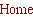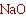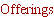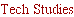Tech Design.....
___________________________

A different look at the Duelund crossover and beyond....
 John KreskovskyMusic and Design© November, 2008
In  Duelund presents a means of developing a crossover for s 3-way speaker system from a unique
approach with result that all the filter functions remain in phase at all frequencies. For details the reader is
referred to the web site where Steen Duelund’s
crossover transfer function for the complete system as
where s is the complex frequency, j ω/ωo , and  ωo is the center frequency of the bandpass response in
a” is the inverse of the damping factor, a = 1/Qd. The crossover has the characteristic that
when separated into HP, BP and LP sections, all sections have the same phase response. While this looks
imposing it is actually very simple. Some clarity can be gained by retaining the denominator in factored
form:
What we have here is a transfer function for which the denominator is the square on a 2nd order, all pole
low pass filter. We can then rewrite this transfer function as the sum of a high pass, band pass and low
pass filters:

H(s) = HP(s) + BP(s) + LP(s)

where
We see further that the HP and LP sections are just cascades of two 2nd order HP or LP filters with the
same Q and the bandpass is a cascade of one each of the 2nd order LP and HP sections with inverted
polarity and gain, G = (1/Q
d)2 – 2.

As Duelund points out, when Q
d = 1/√2, the 2nd order filters are of the Butterworth type and the HP and
LP section are Butterworth squared filters, and form the well known 4th order Linkwitz/Riley crossover.
Note that when Q
d = 1/√2, the bandpass filter has zero gain. Thus, we have a 2-way LR4 crossover for Qd
= 1/√2. Duelund also looks at various other values for Q
d and discusses then in sufficient detail that I will
not repeat it here.

However, it may be of interest to look at what happens when Q
d is 0.5 or less. When Qd is 0.5 or less the
roots of P(s),
The significance of this is that the resulting 4th order transfer function has four poles which are given as
two set of two identical poles. As stated above, when a 2nd order transfer function has two identical poles
Q must equal 0.5. Thus we can rewrite the form of the LP filter as
where

γ = ω1o = ωo2

ω1 being the greater that ωo. That is, for any value of Qd less than or equal to 0.5 the Duelund filter
sections can be expressed as two cascaded 2nd order filters with Q = 0.5 and staggered corner
frequencies ω
1, ω2. In case you haven’t made the connection, since Q = 0.5 yields a 2nd order
d less that or equal to 0.5 the Duelund 3-way crossover HP, LP and BP section
reduce to cascaded 2nd order Linkwitz/Riley filters with staggered cut off frequencies, ω
1 and ω2.

We can thus write the HP and BP sections in this form as well, and repeating the LP section for
completeness we have:
All that remains is to relate G and γ to the Duelund parameters, Qd, and ωo. To that end we find the
following relationships useful:

ωo = √( ω1× ω2)

γ = √( ω
1/ ω2)

G = 1 + 1/γ4

Q
d = γ / (γ2 + 1)

This form is appropriate for all γ ≥ 1.0. The benefit of this cascaded 2nd order Linkwitz/Riley form should be
obvious; we can choose the woofer/midrange, midrange/tweeter crossover points, ω
1, ω2, directly and then
construct the filters, setting the band pass gain factor from knowledge of γ. The band pass gain factor and
Q
d as a function of γ is shown below.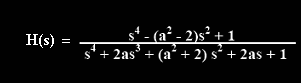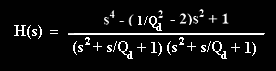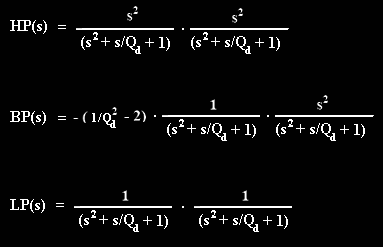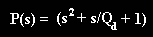are both real numbers. These roots of the denominator of the basic 2nd order transfer function form the
transfer functions poles. These roots correspond to frequencies given as

ω1,2 = ωo [1/(2Qd) +/– Sqrt((1/2Qd)2 -1)]

where the plus sign is used for ω1 and the minus for ω2. These poles characterize the behavior of the filter
response. We note that for Q
d = 0.5, ω1,2 are real and equal, and when Qd is less than 0.5 there are two
unique, real values for ω
1,2, one above ωo and one below ωo.

This leads to an interesting observation. Looking at the LP transfer function we see that there are a total
of four poles, 2 each form each 2nd order section, as indicated below. Each section as the same poles so
that of the four, at most only two are unique. When Q
d is less than 0.5, the poles are all real as was stated
previously.Band pass gain factor and Qd vs. γ for reformulated Duelund crossover.

It should be noted that while Duelund goes to the trouble of defining formulas for passive crossover
components for the filter sections, if the characteristic of the Duelund crossover that all drivers being in
phase at all frequencies, then there is little point of these ideal filter component values. Rather, the transfer
functions should be used as the acoustic targets for LP, BP and HP drivers and the filters developed to
achieve these results. In one considers the midrange driver we see further that given ω
2, the midrange to
woofer crossover frequency, then what is really needed is a sealed midrange enclosure which yields a Q
tc =
0.5 at ω
2. In this regard, the T/S parameters of the midrange driver will dictate the mid/woofer crossover
point. Additionally, the acoustic output of a tweeter is basically that of a 2nd order HP response. Thus to
achieve the Duelund HP acoustic response the poles of the tweeter response must be shifted to that of
either HP section of the cascaded Duelund filter with an additional electrical filter performing the function of
the second section. Similar consideration must be given to the LP response of the woofer and midrange,
taking the acoustic roll off of the midrange driver and woofer into consideration. One important note should
be emphasized. Since the midrange should, in theory, run without a HP filter in a Q = 0.5 sealed enclosure,
there is a danger of over driving the midrange leading to higher distortion and even failure.

Without passing judgment one must wonder as to the benefits of theoretically having all drivers in phase at
all frequencies. The figure below shows an overlay of a 3-way using the Duelund crossover with x-o points at
300 and 2k Hz (γ = 2.582) compared with a 3-way using all LR2 filters. The vertical scale is 5dB/dividion.
Below that are the theoretical 50 Hz square wave response for each, Red = woofer, Green = midrange, Blue
= tweeter and violet = system. Neither shows ringing in the system response while both show some
overshoot in the response of the midrange and tweeter. Additionally, the early time response is not a
monotonic rise in either case.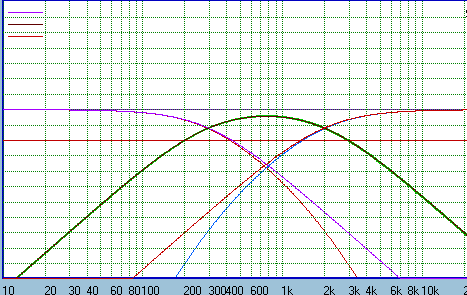Over lay of Duelund 3-way target response with LR2 3-way targets for γ = 2.582 with  ωo= 1k Hz.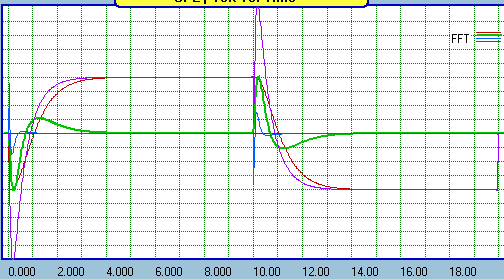50 Hz square wave response of Duelund 3-way filters. Red = LP, Green = BP, Blue = HP and Violet = summed.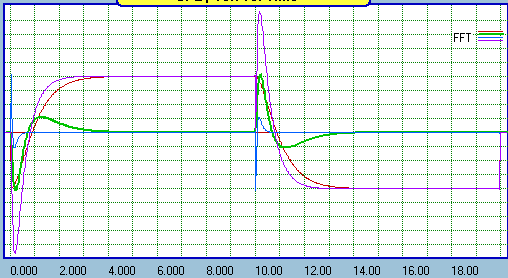50 Hz square wave response of LR2 3-way filters. Red = LP, Green = BP, Blue = HP and Violet = summed.
Below we see the step response for the case of γ = 1.1 on an expanded time scale. Again, Red = LP, green
= BP, Blue = HP and Violet = system. The results are similar to the 50 Hz square wave response. While there
is no overshoot, the rise to the step is not monotonic. There is an initial rise from the tweeter followed by a
dip with contributions from the tweeter and midrange, followed by the rise to the final step amplitude. The
group delay is shown below the step response.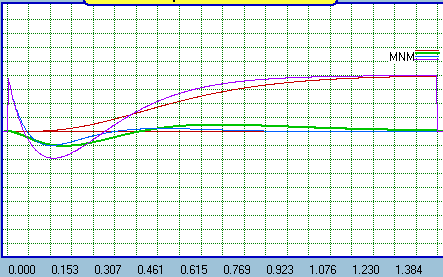Step response of Duelund 3-way crossover. Red = LP, Green = BP, Blue = HP and Violet = summed.
Beyond Duelund

The discussion above demonstrated that for Qd less than or equal to 0.5 the Duelund 3-way crossover
can be constructed from staggered corner frequency, cascaded, 2nd order HP and LP Linkwitz/Riley
transfer functions. All section have two pairs of identical poles; one pair at the low pass and one pair at
the high pass crossover points. I independently found this approach to 3-way crossovers a number of
years ago when investigation the B&O filler driver approach to transient perfect crossover. I did not make
the connection to the Duelund crossover until recently. However, this concept does not stop here. In fact,
3-way crossovers with higher band pass slopes can be developed using the same approach. Not all such
crossovers have the property that all transfer functions have the same phase vs. frequency. The
generalized form of the crossover can be expressed as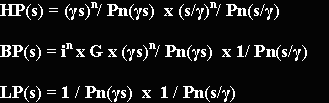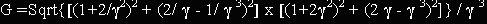and is shown below.
Here γ ≥ 1 and n is the order of the filter polynomial, Pn(), which defines the denominator.  These
expressions show that the bandpass roll off asymptote is always ½ the order of the HP and LP asymptotic
slopes.  N = 1 is, of course, a subset of the generalized, transient perfect, B&O filler driver approach. All
drivers are in phase only when n is an even number. When n is even Pn( ) is of the Linkwitz/Riley type
and the polarity of the band pass is determined by in where i is the square root of -1. Thus for n = 2, as
discussed at length above, the band pass polarity is inverted. When n = 4, the band pass polarity is
positive. When n is odd the band pass sums in quadrature, Butterworth polynomials are used for Pn( ),
and the band pass may be of positive or negative polarity.

In all cases we see that all three sections have two sets of poles, one set each associated with Pn(γs)
and Pn(s/γ).

Example for N = 3

When n = 3 a 3rd order Butterworth polynomial is user for Pn( ). The filters sum in quadrature and a 90
degree phase difference is maintained at all frequencies. It is of interest to note that when γ = 1 the HP
and LP transfer function collapse to LR 6th response. If the HP and LP sections are connected with
normal polarity, a null will occur at the crossover point for any value of γ >1. This null can be “filled” by
the midrange driver. Alternatively, when γ =1 the polarity of the HP or LP section can be inverted yielding
a 2-way, LR6 crossover. The response plot below shows the case for γ = 2.582 (300 Hz and 2k Hz
crossover points). The scale has been changed to 10dB/division to show the change in slope of the HP
and LP section at the LP and HP crossover points, respectively.

The gain for the BP stage is given as
Gain vs. Gamma for N =3
Below the plot of the amplitude response the 50 Hz square wave response when the bands pass is
connected with normal and inverted polarity is shown. While the three section now sum in phase
quadrature it should be noted that when the band pass polarity is inverted the system square wave (and
step) response is similar to the case for n = 2.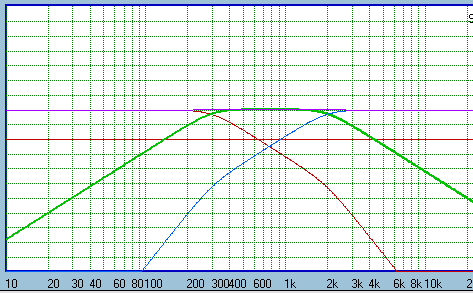Amplitude response for 3-way crossover when n = 3. The band pass
slopes are 3rd order The HP and LP slopes are initially 3rd order
increasing to 6th order outside the band pass corner frequencies.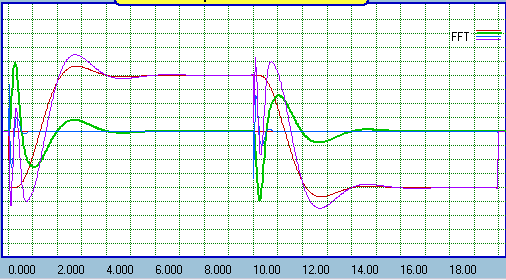50 Hz square wave response for n = 3, in phase band pass.
Note overshoot in system response (violet).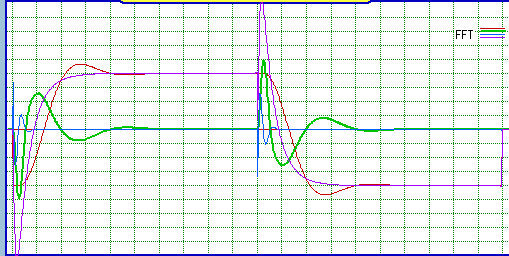50 Hz square wave response for n = 3, inverted band pass.
Note the lack of overshoot in system response (violet).
Example for N = 4.

When n = 4, Pn( ), must be of the form of a 4th order Linkwitz/Riley polynomial. The result is that the
asymptotic slope of the HP and LP section is 8th order while the band pass roll off symmetrically at 4th
order. All filters are connected in phase. All driver are filters are connected in phase and all driver  have
the same phase at all frequencies. The gain for γ >1 is given as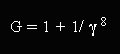and is shown below.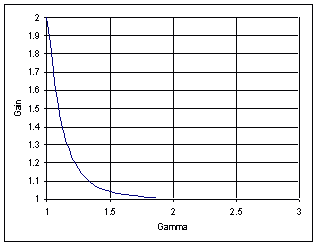Gain vs. Gamma for n = 4.
This filter, like the Duelund 3-way, has all drivers in phase at all frequencies. Also like the Duelund
filter, for = 1 Pn( ) can take on other forms with appropriate adjustment to the BP gain. For example, if
Pn( ) is taken as a 4th order Butterworth polynomial the HP and LP sections default to LR8 filters which
sum flat in phase without the addition of a BP filter. That is, we have a 2-way LR8 crossover. Here we
are concerned only with the case of γ >1.0

The response plot below again shows the case for γ = 2.582 (300 Hz and 2k Hz crossover points). The
scale has again been changed to 10dB/division to show the change in slope of the HP and LP section
at the LP and HP crossover points, respectively. Below the plot of the amplitude response the 50 Hz
square wave response when the bands pass is connected with normal polarity is shown.Amplitude response for 3-way crossover when n = 4. The band pass
slopes are 4rd order. The HP and LP slopes are initially 4rd order
increasing to 8th order outside the band pass corner frequencies.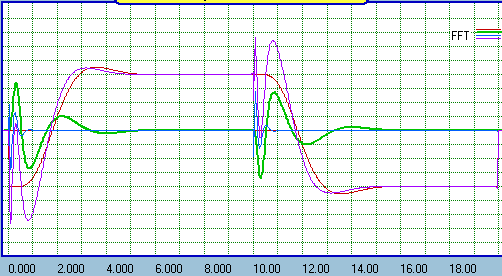50 Hz square wave response for n = 4. Note the slight
overshoot in system response (violet).
1. Duelund, Steen. The art of building a loudsepaker to the end, Part II - The crossover
network. Web publication, circa 2004?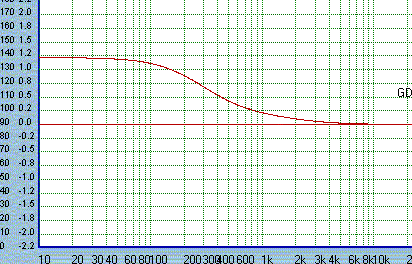Group delay for Duelund 3-way crossover with 300 and 2k hz crossover points.Group delay for n = 3, 3-way crossover with 300 and 2k hz crossover points.
The group delay is identical to that of the Duelund (n=2) crossover.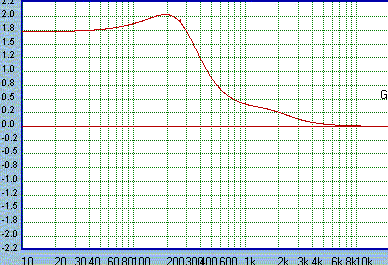Group delay for n = 4, 3-way crossover. Note the higher DC group delay and
non-monotonic rise with bump at 200 Hz, typical of higher order crossovers.